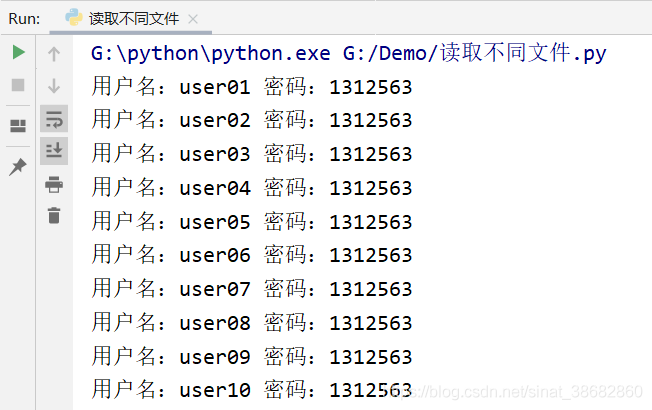﻿ 教你用python将数据写入Excel文件中_python_脚本之家
python# 教你用python将数据写入Excel文件中

Python作为一种脚本语言相较于shell具有更强大的文件处理能力,下面这篇文章主要给大家介绍了关于如何用python将数据写入Excel文件中的相关资料,文中通过实例代码介绍的非常详细,需要的朋友可以参考下

## 一、导入excel表格文件处理函数

```import xlwt
```

## 二、创建excel表格类型文件

```book = xlwt.Workbook(encoding='utf-8',style_compression=0)
```

## 三、在excel表格类型文件中建立一张sheet表单

```sheet = book.add_sheet('豆瓣电影Top250',cell_overwrite_ok=True)
```

## 四、自定义列名

```col = ('电影详情链接','图片链接','影片中文名','影片外国名','评分','评价数','概况','相关信息')
```

## 五、将列属性元组col写进sheet表单中

```for i in range(0,8):
sheet.write(0,i,col[i])
```

## 六、将数据写进sheet表单中

```datalist = [['www','www图片','西游记','xiyouji','100分','0人','很好','超级棒'],['www2','www图片2','西游记2','xiyouji2','1000分','1人','很棒','一级棒']]
for i in range(0,2):
data = datalist[i]
for j in range(0,8):
sheet.write(i+1,j,data[j])
```

## 七、保存excel文件

```savepath = 'C:/Users/DELL/Desktop/excel表格.xls'
book.save(savepath)
```

## 附：Python读取Excel文件数据```#读取xls文件,一定要把xlsx后缀改成xls
import xlrd
file_name = xlrd.open_workbook('G:\\info.xls')#得到文件
table =file_name.sheets()#得到sheet页
nrows = table.nrows #总行数
ncols = table.ncols #总列数
i = 0
while i < nrows:
cell = table.row_values(i) #得到数字列数据
ctype = table.cell(i, 1).ctype #得到数字列数据的格式
if ctype == 2 and cell % 1 == 0: #判断是否是纯数字